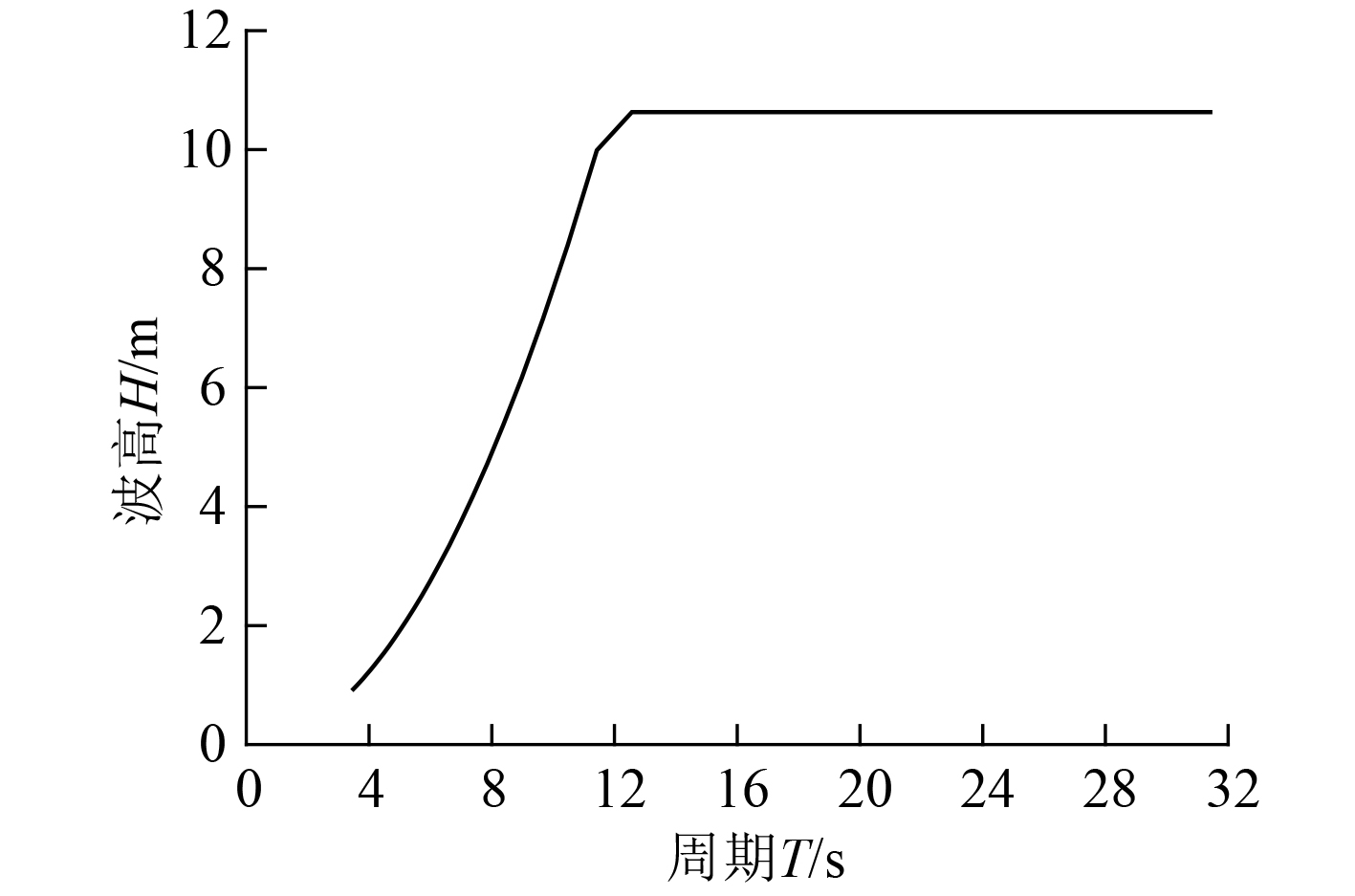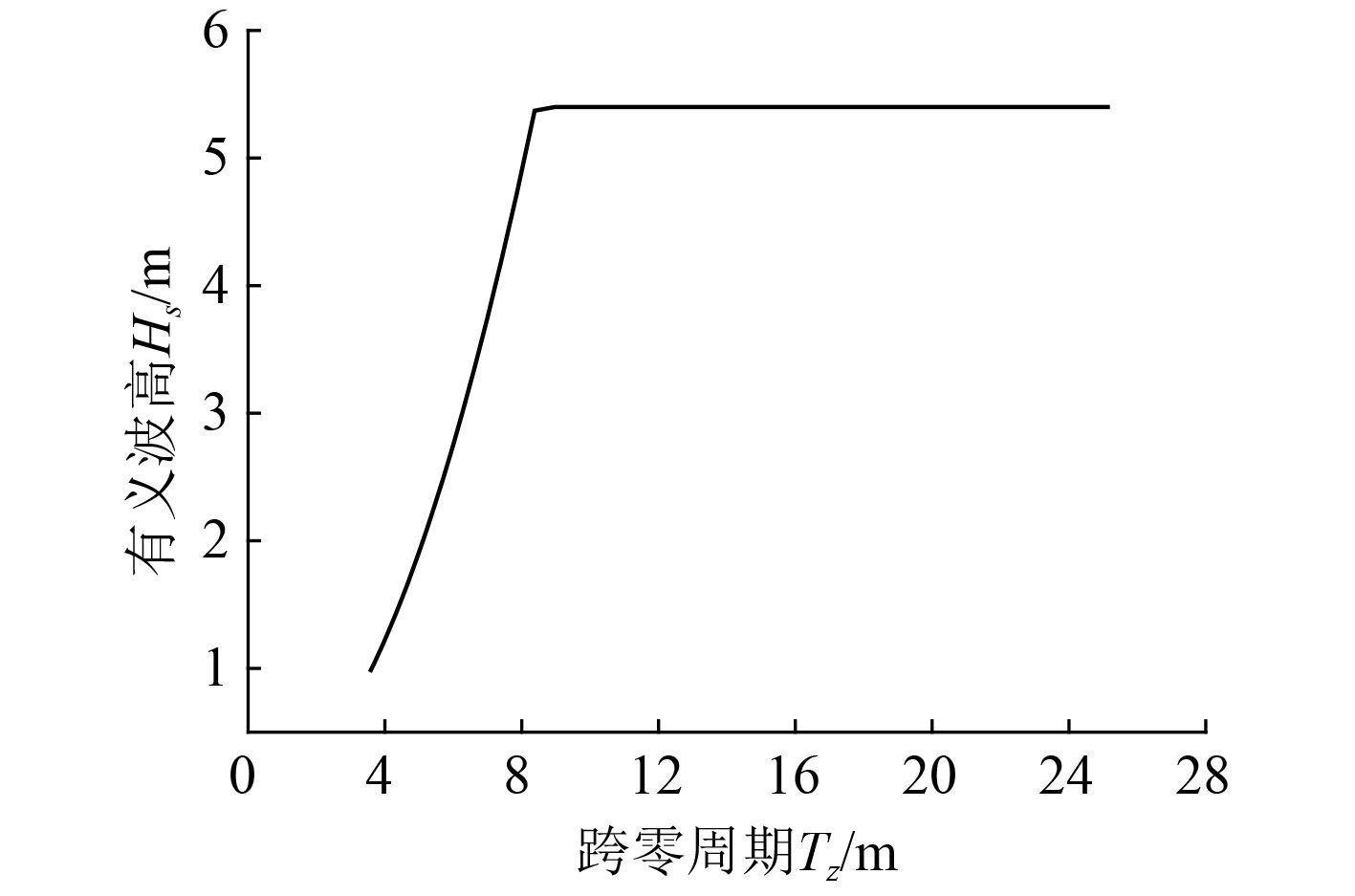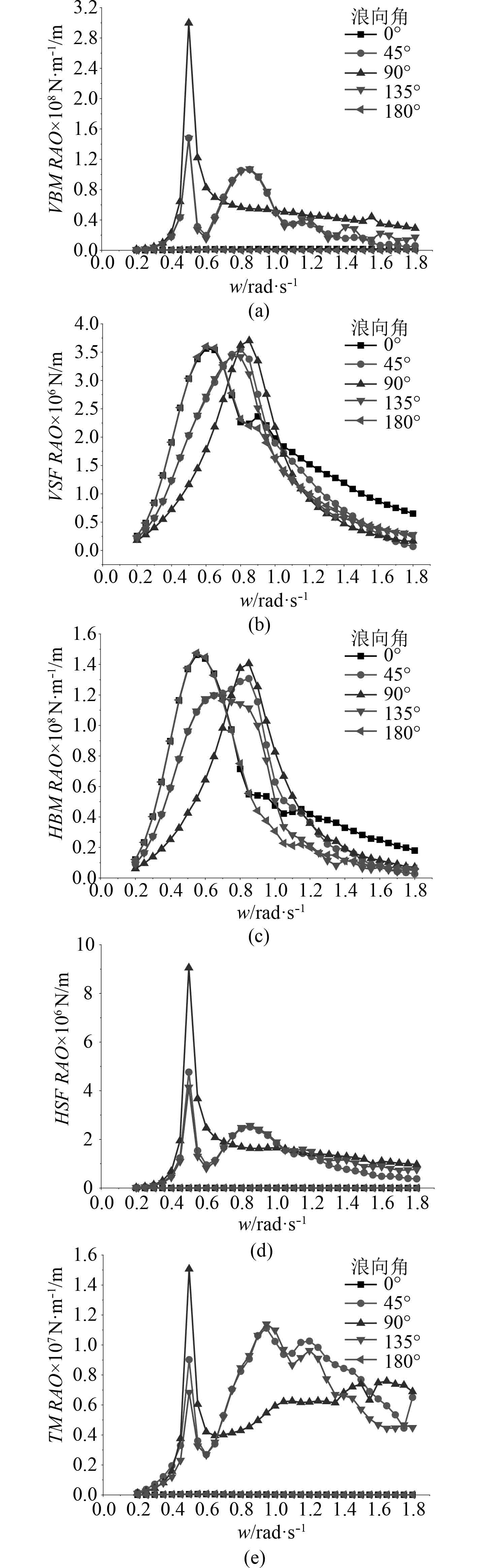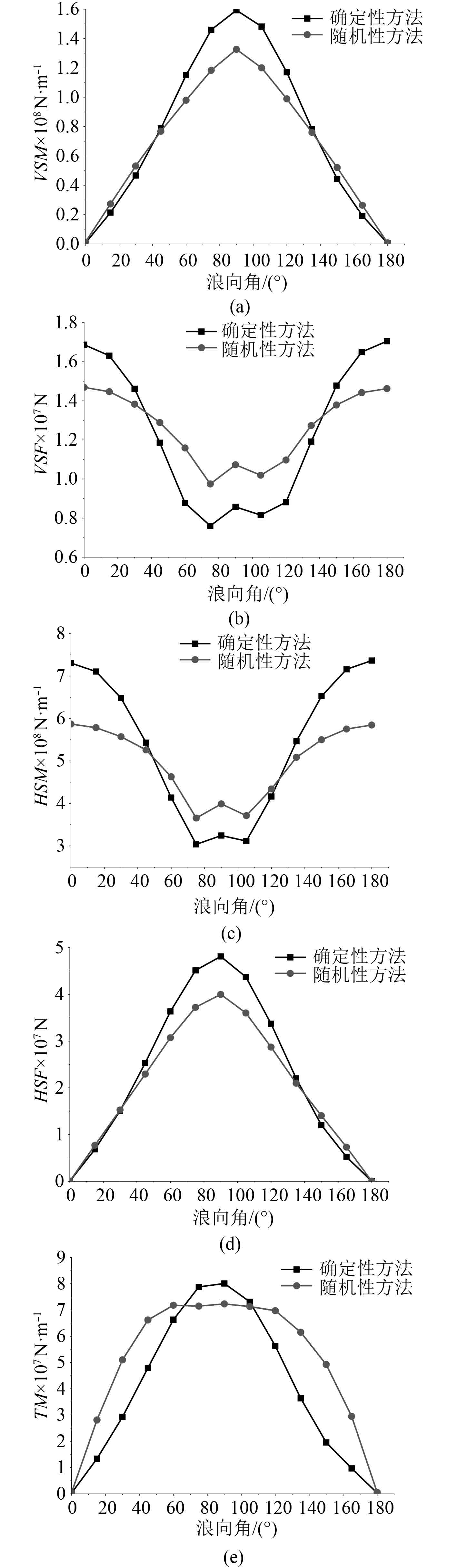﻿ 基于设计波法的船体波浪载荷计算
 舰船科学技术2018, Vol. 40Issue (4): 39-42PDF

Wave loads of ships calculating based on design wave methods
LIANG Shuang-ling, ZHANG Hong-yu, QI Jiang-hui, ZHENG Ya-xiong
Wuhan Second Ship Design and Research Institute, Wuhan 430064, China
Abstract: In this paper, the basic princilpes of the design wave method are discussed and the differences between the different rules in the selection of the dominant load parameters are compared. Deterministic method and stochastic method are used to calculate the wave loads of a ship based on the dominantload parameters of ABS rules. By comparing the design wave parameters and maximum load results of two methods, we can see that deterministic method is conservative in the calculation of the wave loads and stochastic method is more scientific and reasonable due to considering the randomness and irregularity of the wave. The results are helpful for wave loads calculating of similar ships.
Key words: wave loads     design wave method     deterministic method     stochastic method
0 引　言

1 设计波法原理

1.1 载荷控制参数表 1 载荷控制参数 Tab.1 Dominant load parameters表 2 载荷控制参数与评估的船体构件的对应关系 Tab.2 Dominant load parameters and the corresponding structural members
1.2 频率和浪向角

1.3 确定性设计波法

1）由给定范围的频率按照指定波陡确定设计规则波；

2）规则波波幅与该频率对应的载荷响应RAO相乘得到载荷预报值，取载荷预报最大值对应的波高和频率作为设计波参数；

3）浪向角为载荷响应RAO为最大值时对应的浪向角。

 ${S_S} = 2\pi {H_S}/\left( {gT_Z^2} \right)\text{。}$ (1)

 $E\left( {{H_N}} \right) = 2\left( {\sqrt {2\ln N} + \gamma /\sqrt {2\ln N} } \right)\sqrt {{m_0}} \text{，}$ (2)

 ${H_{\max }} = E\left( {{H_{1285}}} \right) = 1.968{H_S} = 10.63\;{\rm{m}}\text{。}$ (3)图 1 设计规则波 Fig. 1 Design regular wave
1.4 随机性设计波法

 ${S_R}\left( \omega \right) = {\left[ {RAO\left( \omega \right)} \right]^2}{S_W}\left( \omega \right)\text{。}$ (4)

 ${R_{\max }} = \sqrt {2\ln N} \sqrt {{m_0}} \text{。}$ (5)

 ${A_D} = \left( {{{R'}_{\max }}/RA{O_{\max }}} \right) \cdot LF\text{。}$ (6)图 2 短期海况 Fig. 2 Short-term wave condition
2 计算结果与分析 2.1 目标船体与计算工况图 3 FPSO水动力模型 Fig. 3 Hydrodynamic model of FPSO
2.2 计算结果 2.2.1 载荷响应RAO图 4 载荷响应RAO Fig. 4 Load response RAO
2.2.2 最大预报载荷图 5 不同浪向角下的最大载荷 Fig. 5 Maximum load in different wave directions
2.2.3 设计波结果表 3 确定性方法结果 Tab.3 Results of deterministic method表 4 随机性方法结果 Tab.4 Results of stochastic method
2.3 结果分析

1）确定性方法得到的设计波波高和最大载荷大于随机性方法，这符合计算越准确，计算结果越小的趋势。

2）确定性方法得到的设计波频率不大于随机性方法，是因为在载荷响应RAO的最大值附近，随着RAO减小而波高有所增大，最大载荷和对应频率发生变化。

3）由2种方法计算得到的设计波浪向角和最大载荷对应的剖面位置相同。

3 结　语

1）由确定性方法和随机性方法计算得到的设计波参数结果相近，作为众多规范推荐的比较成熟的船体波浪载荷计算方法，具有较高的可信度；

2）确定性方法步骤简单，计算得到的设计波波高和最大载荷较大，在船体波浪载荷计算上偏于保守；

3）随机性方法考虑了海浪的随机性和不规则性，在计算原理上更加科学合理。

  张朝阳, 刘俊, 白艳彬. 深水半潜平台波浪载荷计算的设计波方法研究[J]. 中国海洋平台, 2012, 27(5): 34–40. http://www.doc88.com/p-9126121600992.html  ABS. Rules for building and classing mobile offshore drilling unit[S]. 2008.  DNV. Column-stabilized units[S]. 2010.  冯国庆, 任慧龙. 船体结构疲劳评估的设计波法[J]. 哈尔滨工程大学学报, 2005, 26(4): 430–434.  中国船级社. 钢质海船入级规范[S]. 北京: 人民交通出版社, 2015.